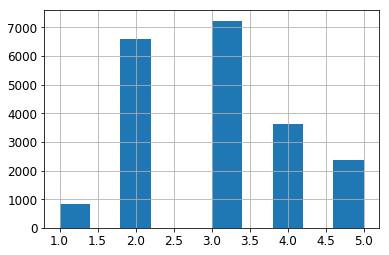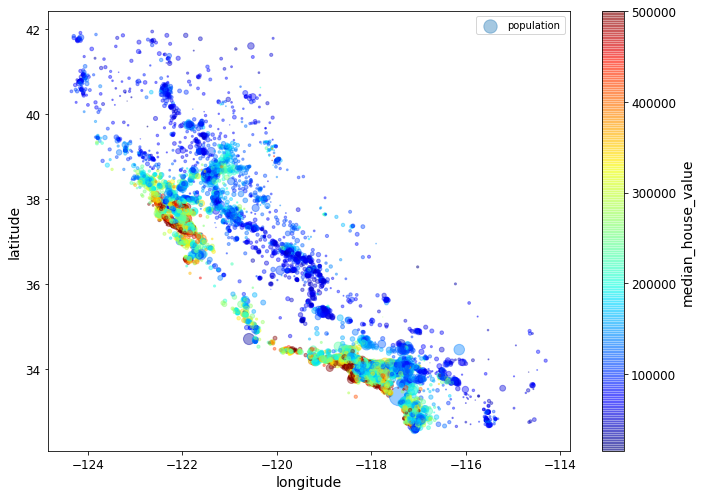# Complete basic project

Handson/ml-2 Aurelien Geron

Chapter 2 – End-to-end Machine Learning project

Welcome to Machine Learning Housing Corp.! Your task is to predict median house values in Californian districts, given a number of features from these districts.

This notebook contains all the sample code and solutions to the exercices in chapter 2.

Note: You may find little differences between the code outputs in the book and in these Jupyter notebooks: these slight differences are mostly due to the random nature of many training algorithms: although I have tried to make these notebooks' outputs as constant as possible, it is impossible to guarantee that they will produce the exact same output on every platform. Also, some data structures (such as dictionaries) do not preserve the item order. Finally, I fixed a few minor bugs (I added notes next to the concerned cells) which lead to slightly different results, without changing the ideas presented in the book.

# Setup¶

First, let's make sure this notebook works well in both python 2 and 3, import a few common modules, ensure MatplotLib plots figures inline and prepare a function to save the figures:

In :
```# To support both python 2 and python 3
from __future__ import division, print_function, unicode_literals

# Common imports
import numpy as np
import os

# to make this notebook's output stable across runs
np.random.seed(42)

# To plot pretty figures
%matplotlib inline
import matplotlib as mpl
import matplotlib.pyplot as plt
mpl.rc('axes', labelsize=14)
mpl.rc('xtick', labelsize=12)
mpl.rc('ytick', labelsize=12)

# Where to save the figures
PROJECT_ROOT_DIR = "."
CHAPTER_ID = "end_to_end_project"
IMAGES_PATH = os.path.join(PROJECT_ROOT_DIR, "images", CHAPTER_ID)

def save_fig(fig_id, tight_layout=True, fig_extension="png", resolution=300):
path = os.path.join(IMAGES_PATH, fig_id + "." + fig_extension)
print("Saving figure", fig_id)
if tight_layout:
plt.tight_layout()
plt.savefig(path, format=fig_extension, dpi=resolution)

# Ignore useless warnings (see SciPy issue #5998)
import warnings
warnings.filterwarnings(action="ignore", message="^internal gelsd")
```

# Get the data¶

In :
```import os
import tarfile
from six.moves import urllib

HOUSING_PATH = os.path.join("datasets", "housing")

def fetch_housing_data(housing_url=HOUSING_URL, housing_path=HOUSING_PATH):
os.makedirs(housing_path, exist_ok=True)
tgz_path = os.path.join(housing_path, "housing.tgz")
urllib.request.urlretrieve(housing_url, tgz_path)
housing_tgz = tarfile.open(tgz_path)
housing_tgz.extractall(path=housing_path)
housing_tgz.close()
```
In :
```fetch_housing_data()
```
In :
```import pandas as pd

csv_path = os.path.join(housing_path, "housing.csv")
```
In :
```housing = load_housing_data()
```
Out:
longitude latitude housing_median_age total_rooms total_bedrooms population households median_income median_house_value ocean_proximity
0 -122.23 37.88 41.0 880.0 129.0 322.0 126.0 8.3252 452600.0 NEAR BAY
1 -122.22 37.86 21.0 7099.0 1106.0 2401.0 1138.0 8.3014 358500.0 NEAR BAY
2 -122.24 37.85 52.0 1467.0 190.0 496.0 177.0 7.2574 352100.0 NEAR BAY
3 -122.25 37.85 52.0 1274.0 235.0 558.0 219.0 5.6431 341300.0 NEAR BAY
4 -122.25 37.85 52.0 1627.0 280.0 565.0 259.0 3.8462 342200.0 NEAR BAY
In :
```housing.info()
```
```<class 'pandas.core.frame.DataFrame'>
RangeIndex: 20640 entries, 0 to 20639
Data columns (total 10 columns):
longitude             20640 non-null float64
latitude              20640 non-null float64
housing_median_age    20640 non-null float64
total_rooms           20640 non-null float64
total_bedrooms        20433 non-null float64
population            20640 non-null float64
households            20640 non-null float64
median_income         20640 non-null float64
median_house_value    20640 non-null float64
ocean_proximity       20640 non-null object
dtypes: float64(9), object(1)
memory usage: 1.6+ MB
```
In :
```housing["ocean_proximity"].value_counts()
```
Out:
```<1H OCEAN     9136
INLAND        6551
NEAR OCEAN    2658
NEAR BAY      2290
ISLAND           5
Name: ocean_proximity, dtype: int64```
In :
```housing.describe()
```
Out:
longitude latitude housing_median_age total_rooms total_bedrooms population households median_income median_house_value
count 20640.000000 20640.000000 20640.000000 20640.000000 20433.000000 20640.000000 20640.000000 20640.000000 20640.000000
mean -119.569704 35.631861 28.639486 2635.763081 537.870553 1425.476744 499.539680 3.870671 206855.816909
std 2.003532 2.135952 12.585558 2181.615252 421.385070 1132.462122 382.329753 1.899822 115395.615874
min -124.350000 32.540000 1.000000 2.000000 1.000000 3.000000 1.000000 0.499900 14999.000000
25% -121.800000 33.930000 18.000000 1447.750000 296.000000 787.000000 280.000000 2.563400 119600.000000
50% -118.490000 34.260000 29.000000 2127.000000 435.000000 1166.000000 409.000000 3.534800 179700.000000
75% -118.010000 37.710000 37.000000 3148.000000 647.000000 1725.000000 605.000000 4.743250 264725.000000
max -114.310000 41.950000 52.000000 39320.000000 6445.000000 35682.000000 6082.000000 15.000100 500001.000000
In :
```%matplotlib inline
import matplotlib.pyplot as plt
housing.hist(bins=50, figsize=(20,15))
save_fig("attribute_histogram_plots")
plt.show()
```
```Saving figure attribute_histogram_plots
```In :
```# to make this notebook's output identical at every run
np.random.seed(42)
```
In :
```import numpy as np

# For illustration only. Sklearn has train_test_split()
def split_train_test(data, test_ratio):
shuffled_indices = np.random.permutation(len(data))
test_set_size = int(len(data) * test_ratio)
test_indices = shuffled_indices[:test_set_size]
train_indices = shuffled_indices[test_set_size:]
return data.iloc[train_indices], data.iloc[test_indices]
```
In :
```train_set, test_set = split_train_test(housing, 0.2)
print(len(train_set), "train +", len(test_set), "test")
```
```16512 train + 4128 test
```
In :
```from zlib import crc32

def test_set_check(identifier, test_ratio):
return crc32(np.int64(identifier)) & 0xffffffff < test_ratio * 2**32

def split_train_test_by_id(data, test_ratio, id_column):
ids = data[id_column]
in_test_set = ids.apply(lambda id_: test_set_check(id_, test_ratio))
return data.loc[~in_test_set], data.loc[in_test_set]
```

The implementation of `test_set_check()` above works fine in both Python 2 and Python 3. In earlier releases, the following implementation was proposed, which supported any hash function, but was much slower and did not support Python 2:

In :
```import hashlib

def test_set_check(identifier, test_ratio, hash=hashlib.md5):
return hash(np.int64(identifier)).digest()[-1] < 256 * test_ratio
```

If you want an implementation that supports any hash function and is compatible with both Python 2 and Python 3, here is one:

In :
```def test_set_check(identifier, test_ratio, hash=hashlib.md5):
return bytearray(hash(np.int64(identifier)).digest())[-1] < 256 * test_ratio
```
In :
```housing_with_id = housing.reset_index()   # adds an `index` column
train_set, test_set = split_train_test_by_id(housing_with_id, 0.2, "index")
```
In :
```housing_with_id["id"] = housing["longitude"] * 1000 + housing["latitude"]
train_set, test_set = split_train_test_by_id(housing_with_id, 0.2, "id")
```
In :
```test_set.head()
```
Out:
index longitude latitude housing_median_age total_rooms total_bedrooms population households median_income median_house_value ocean_proximity id
8 8 -122.26 37.84 42.0 2555.0 665.0 1206.0 595.0 2.0804 226700.0 NEAR BAY -122222.16
10 10 -122.26 37.85 52.0 2202.0 434.0 910.0 402.0 3.2031 281500.0 NEAR BAY -122222.15
11 11 -122.26 37.85 52.0 3503.0 752.0 1504.0 734.0 3.2705 241800.0 NEAR BAY -122222.15
12 12 -122.26 37.85 52.0 2491.0 474.0 1098.0 468.0 3.0750 213500.0 NEAR BAY -122222.15
13 13 -122.26 37.84 52.0 696.0 191.0 345.0 174.0 2.6736 191300.0 NEAR BAY -122222.16
In :
```from sklearn.model_selection import train_test_split

train_set, test_set = train_test_split(housing, test_size=0.2, random_state=42)
```
In :
```test_set.head()
```
Out:
longitude latitude housing_median_age total_rooms total_bedrooms population households median_income median_house_value ocean_proximity
20046 -119.01 36.06 25.0 1505.0 NaN 1392.0 359.0 1.6812 47700.0 INLAND
3024 -119.46 35.14 30.0 2943.0 NaN 1565.0 584.0 2.5313 45800.0 INLAND
15663 -122.44 37.80 52.0 3830.0 NaN 1310.0 963.0 3.4801 500001.0 NEAR BAY
20484 -118.72 34.28 17.0 3051.0 NaN 1705.0 495.0 5.7376 218600.0 <1H OCEAN
9814 -121.93 36.62 34.0 2351.0 NaN 1063.0 428.0 3.7250 278000.0 NEAR OCEAN
In :
```housing["median_income"].hist()
```
Out:
`<matplotlib.axes._subplots.AxesSubplot at 0x114f7c828>`Warning: in the book, I did not use `pd.cut()`, instead I used the code below. The `pd.cut()` solution gives the same result (except the labels are integers instead of floats), but it is simpler to understand:

```# Divide by 1.5 to limit the number of income categories
housing["income_cat"] = np.ceil(housing["median_income"] / 1.5)
# Label those above 5 as 5
housing["income_cat"].where(housing["income_cat"] < 5, 5.0, inplace=True)
```
In :
```housing["income_cat"] = pd.cut(housing["median_income"],
bins=[0., 1.5, 3.0, 4.5, 6., np.inf],
labels=[1, 2, 3, 4, 5])
```
In :
```housing["income_cat"].value_counts()
```
Out:
```3    7236
2    6581
4    3639
5    2362
1     822
Name: income_cat, dtype: int64```
In :
```housing["income_cat"].hist()
```
Out:
`<matplotlib.axes._subplots.AxesSubplot at 0x12b945588>`In :
```from sklearn.model_selection import StratifiedShuffleSplit

split = StratifiedShuffleSplit(n_splits=1, test_size=0.2, random_state=42)
for train_index, test_index in split.split(housing, housing["income_cat"]):
strat_train_set = housing.loc[train_index]
strat_test_set = housing.loc[test_index]
```
In :
```strat_test_set["income_cat"].value_counts() / len(strat_test_set)
```
Out:
```3    0.350533
2    0.318798
4    0.176357
5    0.114583
1    0.039729
Name: income_cat, dtype: float64```
In :
```housing["income_cat"].value_counts() / len(housing)
```
Out:
```3    0.350581
2    0.318847
4    0.176308
5    0.114438
1    0.039826
Name: income_cat, dtype: float64```
In :
```def income_cat_proportions(data):
return data["income_cat"].value_counts() / len(data)

train_set, test_set = train_test_split(housing, test_size=0.2, random_state=42)

compare_props = pd.DataFrame({
"Overall": income_cat_proportions(housing),
"Stratified": income_cat_proportions(strat_test_set),
"Random": income_cat_proportions(test_set),
}).sort_index()
compare_props["Rand. %error"] = 100 * compare_props["Random"] / compare_props["Overall"] - 100
compare_props["Strat. %error"] = 100 * compare_props["Stratified"] / compare_props["Overall"] - 100
```
In :
```compare_props
```
Out:
Overall Stratified Random Rand. %error Strat. %error
1 0.039826 0.039729 0.040213 0.973236 -0.243309
2 0.318847 0.318798 0.324370 1.732260 -0.015195
3 0.350581 0.350533 0.358527 2.266446 -0.013820
4 0.176308 0.176357 0.167393 -5.056334 0.027480
5 0.114438 0.114583 0.109496 -4.318374 0.127011
In :
```for set_ in (strat_train_set, strat_test_set):
set_.drop("income_cat", axis=1, inplace=True)
```

# Discover and visualize the data to gain insights¶

In :
```housing = strat_train_set.copy()
```
In :
```housing.plot(kind="scatter", x="longitude", y="latitude")
```
```Saving figure bad_visualization_plot
```In :
```housing.plot(kind="scatter", x="longitude", y="latitude", alpha=0.1)
save_fig("better_visualization_plot")
```
```Saving figure better_visualization_plot
```The argument `sharex=False` fixes a display bug (the x-axis values and legend were not displayed). This is a temporary fix (see: https://github.com/pandas-dev/pandas/issues/10611). Thanks to Wilmer Arellano for pointing it out.

In :
```housing.plot(kind="scatter", x="longitude", y="latitude", alpha=0.4,
s=housing["population"]/100, label="population", figsize=(10,7),
c="median_house_value", cmap=plt.get_cmap("jet"), colorbar=True,
sharex=False)
plt.legend()
save_fig("housing_prices_scatterplot")
```
```Saving figure housing_prices_scatterplot
```In :
```import matplotlib.image as mpimg
ax = housing.plot(kind="scatter", x="longitude", y="latitude", figsize=(10,7),
s=housing['population']/100, label="Population",
c="median_house_value", cmap=plt.get_cmap("jet"),
colorbar=False, alpha=0.4,
)
plt.imshow(california_img, extent=[-124.55, -113.80, 32.45, 42.05], alpha=0.5,
cmap=plt.get_cmap("jet"))
plt.ylabel("Latitude", fontsize=14)
plt.xlabel("Longitude", fontsize=14)

prices = housing["median_house_value"]
tick_values = np.linspace(prices.min(), prices.max(), 11)
cbar = plt.colorbar()
cbar.ax.set_yticklabels(["\$%dk"%(round(v/1000)) for v in tick_values], fontsize=14)
cbar.set_label('Median House Value', fontsize=16)

plt.legend(fontsize=16)
save_fig("california_housing_prices_plot")
plt.show()
```
```Saving figure california_housing_prices_plot
```In :
```corr_matrix = housing.corr()
```
In :
```corr_matrix["median_house_value"].sort_values(ascending=False)
```
Out:
```median_house_value    1.000000
median_income         0.687160
total_rooms           0.135097
housing_median_age    0.114110
households            0.064506
total_bedrooms        0.047689
population           -0.026920
longitude            -0.047432
latitude             -0.142724
Name: median_house_value, dtype: float64```
In :
```# from pandas.tools.plotting import scatter_matrix # For older versions of Pandas
from pandas.plotting import scatter_matrix

attributes = ["median_house_value", "median_income", "total_rooms",
"housing_median_age"]
scatter_matrix(housing[attributes], figsize=(12, 8))
save_fig("scatter_matrix_plot")
```
```Saving figure scatter_matrix_plot
```In :
```housing.plot(kind="scatter", x="median_income", y="median_house_value",
alpha=0.1)
plt.axis([0, 16, 0, 550000])
save_fig("income_vs_house_value_scatterplot")
```
```Saving figure income_vs_house_value_scatterplot
```In :
```housing["rooms_per_household"] = housing["total_rooms"]/housing["households"]
housing["bedrooms_per_room"] = housing["total_bedrooms"]/housing["total_rooms"]
housing["population_per_household"]=housing["population"]/housing["households"]
```

Note: there was a bug in the previous cell, in the definition of the `rooms_per_household` attribute. This explains why the correlation value below differs slightly from the value in the book (unless you are reading the latest version).

In :
```corr_matrix = housing.corr()
corr_matrix["median_house_value"].sort_values(ascending=False)
```
Out:
```median_house_value          1.000000
median_income               0.687160
rooms_per_household         0.146285
total_rooms                 0.135097
housing_median_age          0.114110
households                  0.064506
total_bedrooms              0.047689
population_per_household   -0.021985
population                 -0.026920
longitude                  -0.047432
latitude                   -0.142724
bedrooms_per_room          -0.259984
Name: median_house_value, dtype: float64```
In :
```housing.plot(kind="scatter", x="rooms_per_household", y="median_house_value",
alpha=0.2)
plt.axis([0, 5, 0, 520000])
plt.show()
```